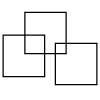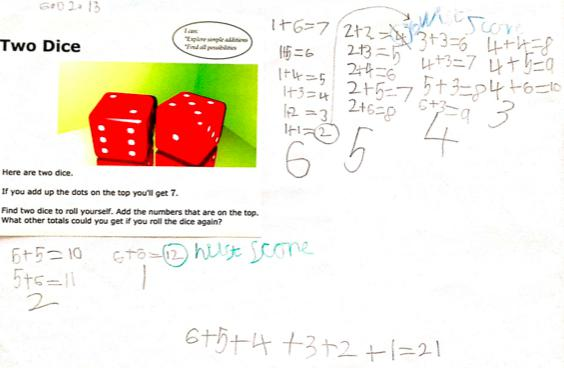#### You may also like### Three Squares

What is the greatest number of squares you can make by overlapping three squares?### Biscuit Decorations

Andrew decorated 20 biscuits to take to a party. He lined them up and put icing on every second biscuit and different decorations on other biscuits. How many biscuits weren't decorated?### Writing Digits

Lee was writing all the counting numbers from 1 to 20. She stopped for a rest after writing seventeen digits. What was the last number she wrote?

# Two Dice

##### Age 5 to 7Challenge Level

It seems that quite a few of you enjoyed the dice rolling needed here.
Joe from Clutton Primary had this work of his commented upon by an adult.

Joe worked systematically to find all the possible combinations for throwing two dice. He identified the lowest possible score of 2 and the highest score of 12. At first he thought that 4 was the lowest score and was then able to correct himself.Robert from St.Michael's Primary West Midlands sent in this very thorough solution;

There are eleven possible solutions to 'Two Dice'.
Let's say Dice One is 'A' and Dice Two is 'B'.

They are as follows :

$2$ (A=$1$ and B=$1$)
$3$ (A=$1$ and B=$2$; A=$2$ and B=$1$)
$4$ (A=$1$ and B=$3$; A=$2$ and B=$2$; A=$3$ and B=$1$)
$5$ (A=$1$ and B=$4$; A=$2$ and B=$3$; A=$3$ and B=$2$; A=$4$ and B=$1$)
$6$ (A=$1$ and B=$5$; A=$2$ and B=$4$; A=$3$ and B=$3$; A=$4$ and B=$2$; A=$5$ and B=$1$)
$7$ (A=$1$ and B=$6$; A=$2$ and B=$5$; A=$3$ and B=$4$; A=$4$ and B=$3$; A=$5$ and B=$2$; A=$6$ and B=$1$)
$8$ (A=$2$ and B=$6$; A=$3$ and B=$5$; A=$4$ and B=$4$; A=$5$ and B=$3$; A=$6$ and B=$2$)
$9$ (A=$3$ and B=$6$; A=$4$ and B=$5$; A=$5$ and B=$4$; A=$6$ and B=$3$)
$10$ (A=$4$ and B=$6$; A=$5$ and B=$5$; A=$6$ and B=$4$)
$11$ (A=$5$ and B=$6$; A=$6$ and B=$5$)
$12$ (A=$6$ and B=$6$)

Two $1-6$ dice will not add up to any other number. This solution shows the
amount of numbers possible to make. However there are 36 possible

Pupils from David Hoy Elementary School worked on the challenge and this was the result;

Step 1: Explore
In groups of three we took turns rolling the dice. Then we recorded our answers, as this was going to help us remember what numbers we found. In some groups everyone wrote down the number sentences, in other groups, each person wrote one of the addends, and the third person wrote the solution.
(Ex: 4+3=7)

Step 2: Making a class list of all the numbers we found and how
We read the numbers we found off of our sheets checking to see if we had rolled the same as other groups, and then looking for other numbers we missed.

Step 3: To make sure we had found all the numbers, the teacher asked what the largest number was and why
"12, it can't be bigger than 12"
"It can't be a very big number cause there is only like 4 maybe 5 sides on the dice, so not many numbers."
"Six was the biggest number on the dice."
"Double six is 12, so that is the biggest number."
"Then the smallest has to be 2, cause double 1 is 2, and 1 is the smallest number."

Teacher: Can we get all the numbers in between?
"Look at the board, and put the solutions on a number line."

Which is what we did, and we discovered that there were multiple ways to get some of the numbers, but only one way to get 2 and 6....  Next I think we will try and figure out how many ways we can get the numbers 3-11 using two dice.

Their teacher said:

What a nice problem! My class loved it, it was a great way to practise counting, counting on, and writing number sentences.

Well done, thank you so much for all the contributions.  Submissions that include some reflections of what happened are always good to share.